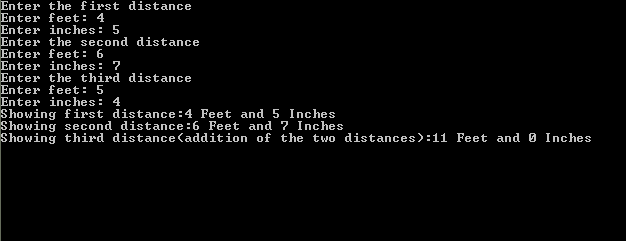# Returning Objects from Function in C++

Returning Objects from Function in C++ In this tutorial you will learn: 1. How to pass an object in a function argument 2. How to return an object from a function 3. How to write functions outside class 4. Basic C++ syntax In C++ when we need to return a variable from a function we have to write its return type before the name of function and arguments. As class can also be thought of as a type of object so in C++ we can also return whole object from a function. Object of class ‘myClass’ can be returned from a function as follow
`myClass returnFunc(){//this class will return an object thorugh the function}`
The functions can be written in the class and outside the class as well according to the wish of the programmer, in this tutorial I have demonstrated how to write the function outside the class. Basic syntax for writing a function outside the class is as follow
`Returntype nameOfTheClassToWhichFunctionBelongs :: nameOfFunction(ArgumentsIfAny){//function definition}`
It must be kept in mind that the function has to be called declared in the class of the program so that main knows that the function exist otherwise the program will not run and error will popup. Program: strong>Code Basic Step: Open Dev C++ then File > new > source file and start writing the code below.
`#include<iostream>#include<conio.h>using namespace std;`
These are the basic header files which are required for C++ programming. Using namespace std is used at the top of the program because if its not written then we have to write ‘std::’ with every line of the program. #include includes the C++ built in libraries and iostream is used for input and output of the program. Conio.h is used to show output on the screen, if it is not present , the screen flashes and disappears hence not showing output.
`public:	Distance(int ft, float in)	{		feet=ft;		inches=in;	}		void setDist()	{	cout<<"Enter feet: ";	cin>>feet;		cout<<"Enter inches: "; 	cin>>inches;}	void showDist() 		{		cout<<feet<<" Feet and "<<inches<<" Inches"<<"\n";	}	Distance addDist(Distance d);};`
In this program we made a class named Distance, which is having two data members that are feet and inches. Firstly in private access specifier we defined the data members and in the next few line we made its overloaded constructor and then defined a function ‘setDist’. This function is basically a setter function which set the values of feet and inches according to the wish of user. The next function we have in our class is the showDist function which is basically a getter function and instead of returning some value it displays the result on the screen. It shows the feet and inches on the screen.In the last line of the class we declared a function ‘addDist’, this function is defined outside the class as shown in code below.
`Distance Distance::addDist(Distance d2){ 	Distance temp(0,0);   	temp.inches=inches + d2.inches;   	  if (temp.inches>=12.0) 	 {		temp.inches -= 12;		temp.feet=1;	}	temp.feet += feet + d2.feet;	return temp;	}`
This is the function that takes in an object of the same class as of the return type as an argument and add the distance(inches and feets) and return another object. It must be noted here that as the object is returned to the main so there must be some object in the main of the same class so the data member of the returned object get stored in the object that is present in main. This function creates an object named ‘temp’. In data members of temp it stores the addition results of corresponding data members of the passed object and the object through which the function is called through main. Finally returns the object ‘temp’.
`void main() {	Distance d1(0,0), d3(0,0); //distances initialized to 0	Distance d2(0,0);	cout<<"Enter the first distance\n";	d1.setDist();	cout<<"Enter the second distance\n"; 	d2.setDist();	cout<<"Enter the third distance\n";	d3.setDist();	d3=d1.addDist(d2);	cout<<"Showing first distance:";	d1.showDist(); 	cout<<"Showing second distance:";	d2.showDist(); 	cout<<"Showing third distance(addition of the two distances):";	d3.showDist();    getch(); }`
In the main of the program we simply take in the inputs by the user , At first the objects are initialized to 0 but in the next lines using setDist function user give inputs so user can have liberty to give distance according to her wish. Then we displayed the result for each object using showDist function and also displayed the result of addition of two objects add the end. Execute > compile then Execute > run Output